Latest Banking jobs   »

# Quantitative Aptitude Quiz For IDBI AM/Executive 2022- 8th June

(a) x < y
(b) x ≤ y
(c) x = y or no relation can be established between x and y.
(d) x > y
(e) x ≥ y

Quantitative Quiz For IDBI AM/Executive 2022- 8th June CHECK IN HINDI

Q1. (i) x²-25x+156=0
(ii) y²-21y+108=0

Q2. (i) 3x + 5y = 18
(ii)7x + 8y = 42

Q3. (i)2x² + 15x + 27 = 0
(ii)3y² + 25y – 18 = 0

Q4. (i)6x² + 29x + 35 =0
(ii)20y² + 27y + 9 =0

Q5. (i)x² – 7x – 30 = 0
(ii)y² + 15y + 50 = 0

Directions (6-10): Given below are two equations in each question, which you have to solve the equation and give answer
(a) if x>y
(b) if x≥y
(c) if y>x
(d) if y≥x
(e) if x=y or no relation can be established

Q6. I. x² – 5x + 4 = 0
II. y² + 3y + 2 = 0

Q7. I. 6x² – 5x + 1 = 0
II. 15y² – 8y + 1 = 0

Q8. I. x² + 5x + 4 = 0
II. y² – 5y = 14

Q9. I. x² + 9x + 20 = 0
II. y² + 4y+ 3 = 0

Q10. I. x² – 6x + 8 = 0
II. y² + 2y = 8

(a) x < y
(b) x ≤ y
(c) x = y or no relation can be established between x and y.
(d) x > y
(e) x ≥ y

Q11. I. 2x² + 21x – 36 = 0
II. 2y² – 15y + 18 = 0

Q12. I. 12x² – 31x + 20 = 0
II. 10y² – 29y + 21 = 0

Q13. I. x² – 39x + 360 = 0
II. y² – 26y + 168 = 0

Q14. I. 4x² – 11x – 38 = 0
II. 3y² – 14y – 69 = 0

Q15. I. 5x + 7y = 24.5
II. 4x + 9y = 40

Solutions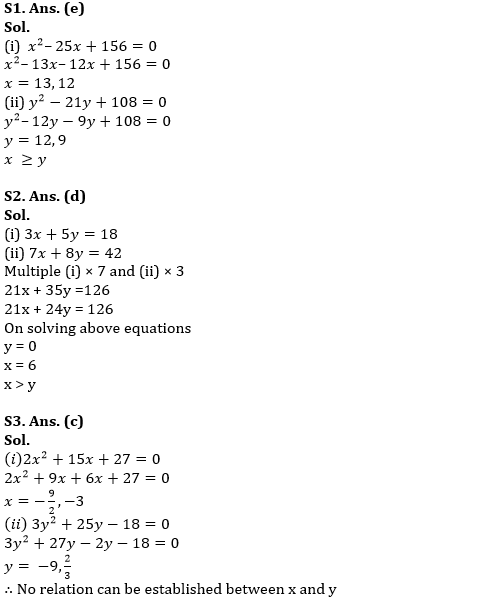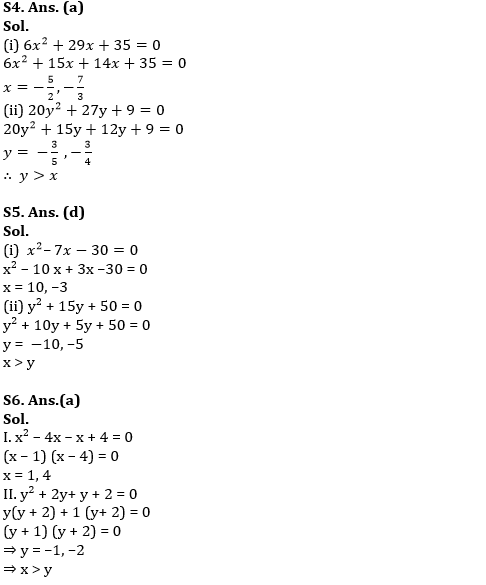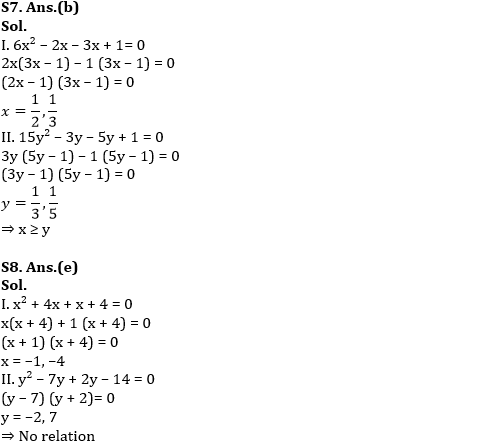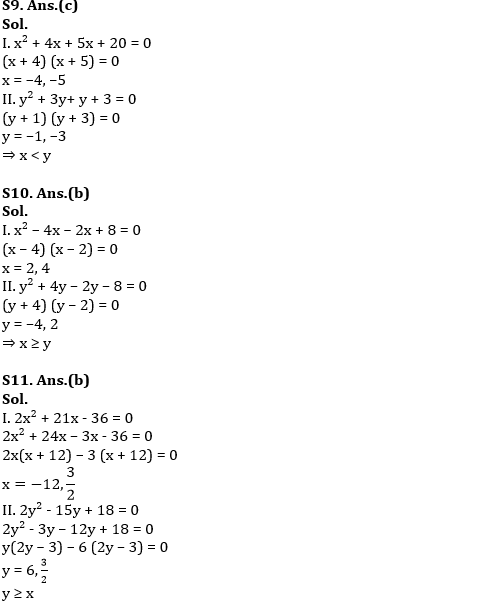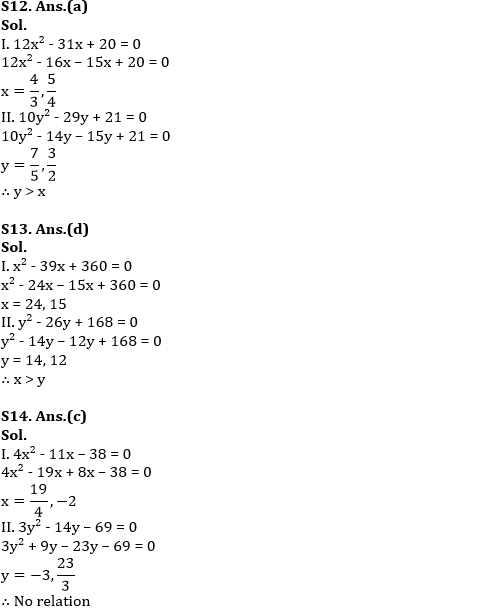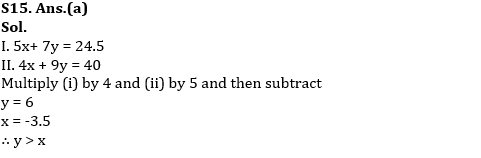#### Congratulations!